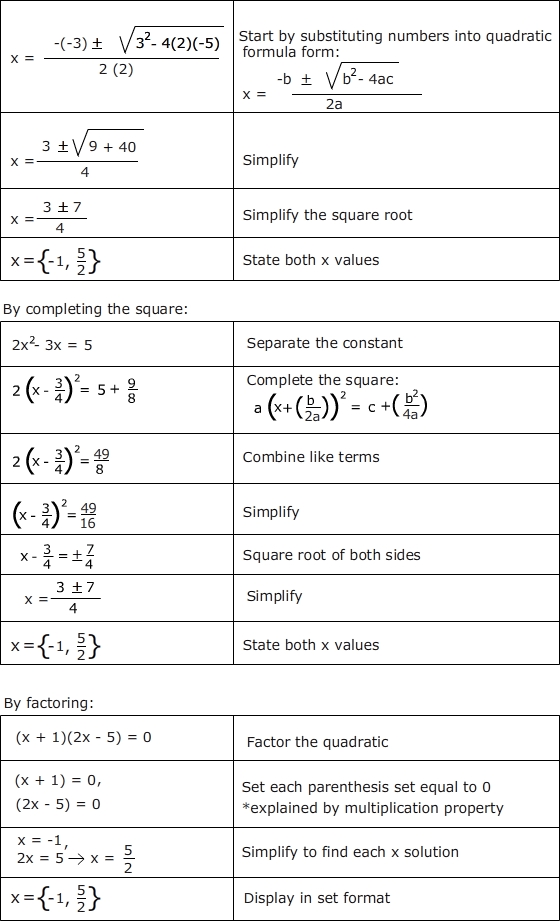# Week 6 – Pre Calculus 11

This week in Pre Calculus 11 we where introduced to three ways of solving a quadratic equation the three types of ways are:

1. Factoring the equation
2. Completing the square